# Teaching a Computer Gamerules

## Sequential games with perfect information

### 1. A very short course in Game Theory

In the twenties people start to describe games using math. Since then Game Theory becomes an important technique in economy and and nowadays, with artificial intelligence becoming more important, even computer silence. We want to follow the usual notation but only give a very short repetition of the most important parts. For a deeper intro into the topic i recommend A primer in Game Theory by Robert Gibbons. To describe a game at first we need a description of the players playing the game, in particular letbe the set of players. The second important information is which rules apply to the game. This is described bywhich is a set of all possible sequences (finite or infinite),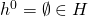is the initial history andthe terminal history. To describe the histories in between we introduce the concept of actions. At each stageof the game the players, say, have to choose an actionfrom the setwhich is the set of actions or strategies available to player. Accordingly,with. Then a history at stageis described bywithbeing the action profile stage 0. Accordingly for,,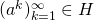if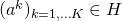for all positive. We also need to determine which players turn is at a certain stage. Letthe player function that determines who is next to the respective sequence,. Finally we need to determine the outcomes of the game byis the set of utility functions. With these information we can now describe a perfect-information extensive-form game as.

### 2. Example: Tic-Tac-Toe

Tic-Tac-Toe is a sequential two player game, who take turns marking the spaces in agrid with eitheror. The player who succeeds in placing three of their marks in a horizontal, vertical, or diagonal row wins the game. If we consider a board with the nine positions numbered as follows:

 1 2 3 4 5 6 7 8 9

We can define the Game bywhere 1 is the Player who playsand 2 the player who plays. The set of all sequencesis then given bywhere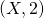is a possible move of Player 1 where the second field is marked anda possible move after by Player 2 and so on. Accordingly for example,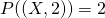. Let(draw, lost) a history where Player 1 wins the game, a possible payout function one can for example define.

### 3. Implementing a game

In the following we want “teach” a computer how to play a game. In particular this requires to let the computer know which movesat particular stageare allowed. As the reader might recognize this information is included in. Even though for simple games like Tic-Tac-Toe it is not difficult for a computer to write down (and therefore implement)in particular (in case of Tic-Tac-Toe we are dealing with 255.168 games), for more complicated games like chess or GO it turns out that (at the current level of computer power) this task is impossible. This information is for example required to let the computer play the best strategy. However, this is not what we want to achieve today. At the moment we only want to construct a computer that understands and plays by the rules without a certain strategy (or more precise: a random strategy).
To let the computer “know” the allowed actions at stagewe introduce a new functionwhich map a given history to all possible actions,. For example. We will make another simplification here. For many games, including Tic-Tac-Toe, Chess or GO, the knowledge of the history is not necessary to make the next move. The only necessary information is the current state of the game. Games where the history is unknown are called “games with complete information” in contrast to “games with perfect information” which describes games where the history is known to each player at each stage. In the following we present a Javascript example where the computer plays Tic-Tac-Toe against himself by randomly choosing cells. The “gamerule()” mapswith “f”,with “reward” andwith “turn”.

At a first look the outcome of running 10.000 random games was surprising. In Table we see a clear first mover advantage. Intuitively I suspected the chances of winning the game to be equal, however after a second thought this result is not surprising because the first mover gets 5 moves as compared to 4 moves for the second player. In particular, according to Wikipedia, there are 255.168 possible games, in 131.184 of these games the first player wins while in 77.904 the second player wins and in 46.080 the game ends with a draw. In the Table we can see that this differs from our estimation. The reason are the very special rules of the Tic-Tac_Toe Game. In fact the game could end earlier if one player succeeds in making the row before the last turn. There are  5328 possibilities for games ending in a win on the sixth move, 47952 possibilities for games ending in a win on the seventh move, 72576 possibilities for games ending in a win on the eighth move and 81792 possibilities for games ending in a win on the ninth move.
Since we play the Game sequentially, a path where the game ends earlier will be reached more often then path where the game ends later. To see this we take a look at a historythat ends afterturns and a path with a historythat ends afterturns. Let’s say we reach the path of each history afterturns 2 times. Since forthis is the final knot, this will count two times for. Forthere are at least two further histories to reach in the next turn and therefore the chance to reach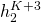iswhich means that we will reachonly half as much as. However, doing a quick Google I was able to find some results ( here, here ) that supports my results.

Outcome Estimated probability Wikipedia probability
Player 1 wins 0.5859 0.5141
Player 2 wins 0.2874 0.3053
Draw 0.1267 0.1806
/*
--Heiko Wagner 2019
*/

class Matrix {
constructor(data, ncols) {
//reshape the data
this.ncols = ncols;
var ncols = ncols;
var data = data;
this.matrix = []
for (var m = 0; m &lt; data.length / ncols; m++) {
var row = []
for (var n = 1; n &lt;= ncols; n++) {
row.push(data[n + (m * ncols) - 1])
}
this.matrix.push(row)
}
}
transpose() {
return new Matrix(this.matrix.map((_, c) =&gt; this.matrix.map(r =&gt; r)).flatMap(x =&gt; x), this.ncols)

}
trace() {
return this.matrix.map((x, i) =&gt; x[i]).reduce((a, b) =&gt; (a === null || b == null) ? null : a + b)
}
rowsums() {
return this.matrix.map((x) =&gt; x.reduce((a, b) =&gt; (a === null || b == null) ? null : a + b))
}
flip() {
var nrows = this.matrix.length
return new Matrix(this.matrix.map((x) =&gt; x.reverse().flatMap(x =&gt; x)).flatMap(x =&gt; x), nrows)
}
}

function getRandomInt(max) {
return Math.floor(Math.random() * Math.floor(max));
}

function gamerules(state, turn, player) {
//check for win else
//win if any rowsum is 3 or 0, trace or flip.trace is 3 or 0
var has_won = [state.rowsums(),
state.transpose().rowsums(),
state.trace(),
state.flip().trace(),
].flatMap(x =&gt; x)

var reward = null;

if (has_won.includes(0)) {
//console.log("0 has won")
if (player == 0) {
reward = 1
} else {
reward = -1
}
}

if (has_won.includes(3)) {
//console.log("1 has won")
if (player == 1) {
reward = 1
} else {
reward = -1
}
}

var state_init = state.matrix.flatMap((x)=&gt;x)

f = []
for (var l = 0; l &lt; state_init.length; l++)
{
var state_l = state_init.slice(0);
if (state_init[l] == null) {
state_l[l] = turn
f.push(new Matrix(state_l,3) )
}
}

//check if no free field are left ==&gt; draw
if (f.length==0 &amp;&amp; reward == null) {
reward = 0
}
return { f: f, reward: reward, turn: (turn + 1) % 2 }
}

//run trough all states of a game until an reward is reached
//play a random game starting from state with gamerules

function random_game(state) {
//play initial turn
var choices = gamerules(state, 1)
while (choices.reward === null) {
var K = choices.f.length
var decision = getRandomInt(K)
choices = gamerules(choices.f[decision], choices.turn, 0)
}
return choices
}

//Intial state of the game
var state = [null, null, null, null, null, null, null, null, null]
test = new Matrix(state, 3)
//Example of \mathcal{H}:
//gamerules(test, 1)

//Play a single random game
random_game(test)

//Play 10000 random games and make a histogram
M=10000
var win =0
var loss = 0
var draw = 0

for (var i=0; i &lt; M; i++)
{
var outcome = random_game(test).reward

switch(outcome) {
case 1:
win++;
break;
case -1:
loss++;
break;
case 0:
draw++;
}
}

var M2=draw+win+loss
console.log("prop of player 1 winning: "+loss/M)
console.log("prop of player 2 winning: "+win/M)
console.log("prop of draw: "+draw/M)



### 1 thought on “Teaching a Computer Gamerules”

This site uses Akismet to reduce spam. Learn how your comment data is processed.